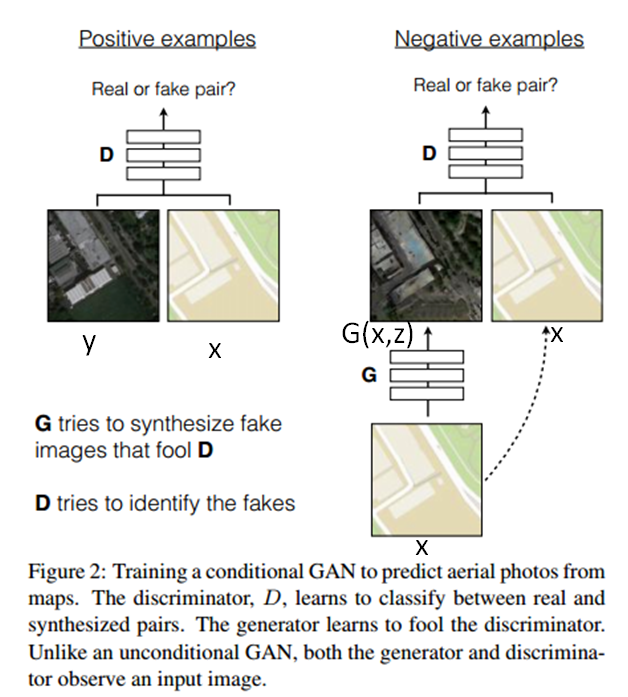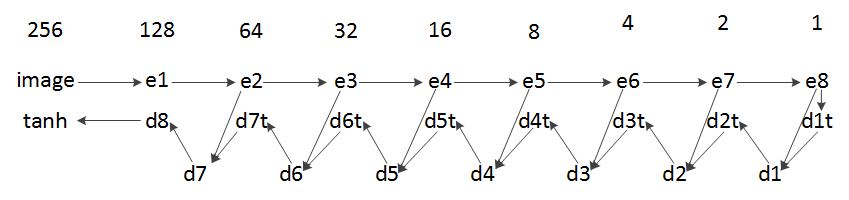## （原）GAN之pix2pix

https://www.cnblogs.com/darkknightzh/p/9175281.html

Image-to-Image Translation with Conditional Adversarial Networks

https://arxiv.org/pdf/1611.07004v1.pdf

pix2pix使用的是Conditional GAN（cGAN）。传统的GAN通过随机向量z学习到图像y：$G:z\to y$；cGAN则是通过输入图像x及随机向量z学到图像y：$G:\{x,z\}\to y$。其目标函数是

${{L}_{cGAN}}(G,D)={{E}_{x,y\sim {{p}_{data}}(x,y)}}\left[ \log D(x,y) \right]+{{E}_{x\sim {{p}_{data}}(x),z\sim {{p}_{z}}(z)}}\left[ \log (1-D(x,G(x,z))) \right]$${{L}_{L1}}(G)={{E}_{x,y\sim {{p}_{data}}(x,y),z\sim {{p}_{z}}(z)}}\left[ {{\left\| y-G(x,z) \right\|}_{1}} \right]$

${{G}^{*}}=\arg \underset{G}{\mathop{\min }}\,\underset{D}{\mathop{\max }}\,{{L}_{cGAN}}(G,D)+\lambda {{L}_{L1}}(G)$

pix2pix未使用传统的encoder-decoder的模式（下图左侧），而是使用了U-Net（下图右侧）网络。U-Net论文为：U-net: Convolutional networks for biomedical image segmentation。U-net在decoder部分，每个conv层之前将输入和decoder对应的镜像层进行了拼接，因而输入的通道数增加了1倍，但是不严谨的说，输入的通道数不会影响卷积的输出维度，因而网络不会出问题。posted on 2018-06-12 22:07  darkknightzh  阅读(17726)  评论(0编辑  收藏  举报